##1. Polar line of a point A with respect to circle c

A thorough understanding of this notion presupposes the knowledge of harmonicity (see Harmonic.html ).

Consider a circle c(E,r) and a point A. On every secant line e =  BA through A define the harmonic conjugate of A with respect to BC, i.e.
point D on e such that: DB/DC = - AB/AC.  Then, for (e) rotating about A the corresponding point D moves on a line (f).
This is the Polar of A with respect to the circle c. A is called the Pol of line (f) with respect to the circle. Besides the polar (f) of point A is
orthogonal to line EA.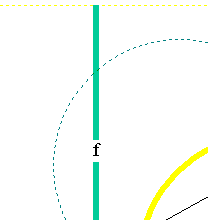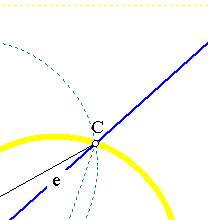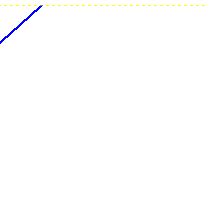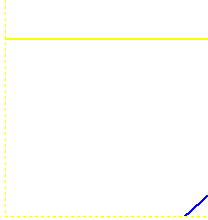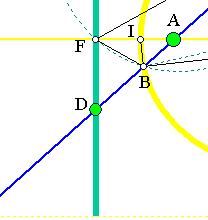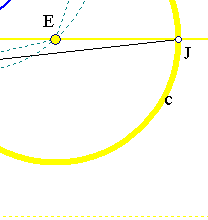Draw the circle through B, C and the center E of circle c(r). This intersects line  AE at F. Triangle BCE is isosceles and this implies that
angle (BFA)=(ACE)=(ABE)=(AFC). Thus line AF is bisector of the angle BFC. Hence point D being harmonic conjugate to A with
respect to {B,C} is on the outer bisector and angle DFA is a right one.
Let IJ be the diameter on AE. Line BI bisects angle ABF. This is seen by first noticing the similarity of triangles BFE and BAE, due
again to the isosceles property of BEC. In fact (BFI)+(FBI)=(BIE) and (IBA)+(ABE)=(IBE)=(BIE). Using (BFI)=(ABE) =>
(FBI)=(IBA) and since IBJ is a right angled triangle points {I,J,A,F} build a harmonic division i.e. F belongs to the polar of A. This
proves all claims.

##2. Reciprocity of the pol-polar relation

The previous definition and proof shows that every line through A intersecting its polar at D and the circle at {B,C} has {B,C} harmonic
conjugate with respect to {A,D}. This is a symmetric relation and implies the so-called pol-polar reciprocity property:
If the polar pA of A passes through D then the polar pD of D passes through A.
In other words this means that all the polars pD of points D on the polar of pA of A pass through A.

Corollary  The intersection point (D) of two polars (e), (f) of two points K and A is the pol of line  KA.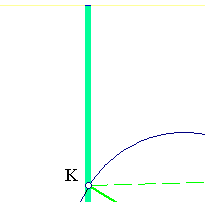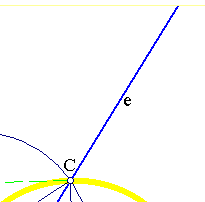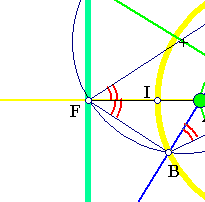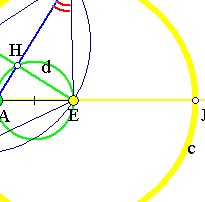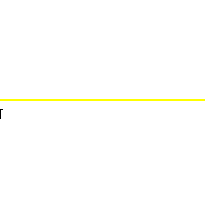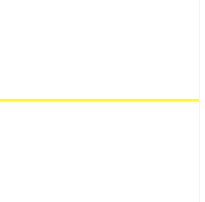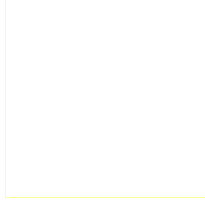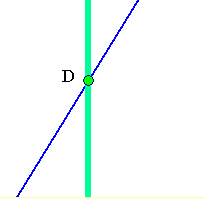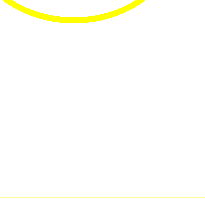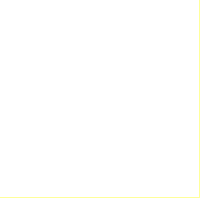##3. Additional properties

Using the above figure.
1) AE*FE = r2, A and F are inverse with respect to c(r). This identifies the polar pA of A with the line (f) orthogonal to AE at F,
which is the inverse of A with respect to c(r).
2) The inverse of the polar (f) with respect to c(E,r) is the circle (d) with diameter AE.
3) Every point K on the the polar (f) is inverted into some point H and by (1) line AH is the polar of I.
4) The polar (f) of point A is the inverse of the circle (d) with diameter AE.
5) Quadrangle KCEF being cyclic, the tangents CK, KB of c(E,r) at B and C meet at the polar of A.
6) For points A lying exactly on the circle c(E,r) the corresponding polar is the tangent at A.
7) By convention the polar of the center E is the line at infinity. Inversely every point at infinity A* determines a
direction (v) of parallel lines and the polar of A* is identified with the line through the center E whose direction
is orthogonal to v. For a practical construction of the polar of a point see the file Polar2.html . Some further properties of the polar are
discussed in the file Polar3.html and Polar4.html .

##4. Tangents and their chord of contacts

Let circle c be tangent to lines {AX, AY} at {B,C} respectively. Then for every point E on the circle the tangent tE intersects
lines {AX, AY, BC} respectively at points {H,F,G} such that {E,G} are harmonic conjugate with respect to {F,H}.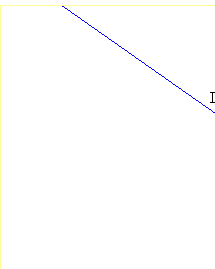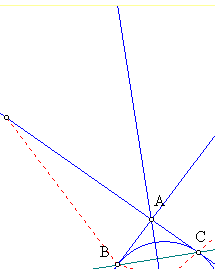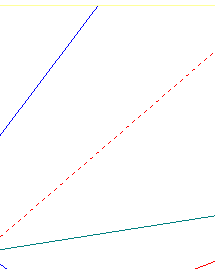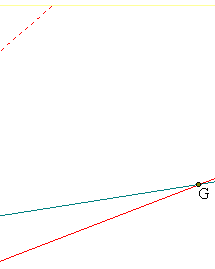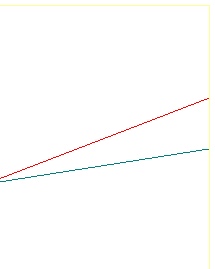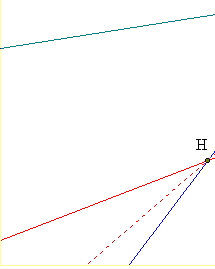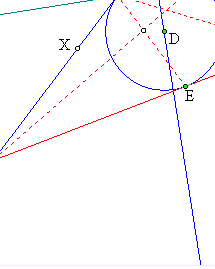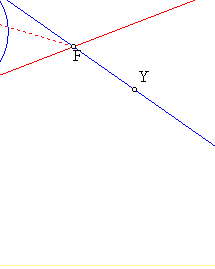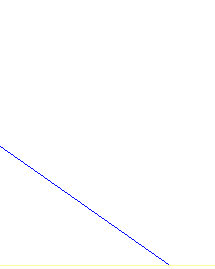Let I be the intersection point of AY with BE which is the polar of H. Then the polar pI of I is CH. This because it contains
H by the pol-polar reciprocity and passes obviously through C. This, using the harmonic pencil at H: H(I,C,A,F), implies that
{A,F} are harmonic conjugate to {C,I}. Hence the pencil of lines at B: B(A,F,C,I) is also harmonic, hence its intersection
with line tE defines a harmonic division (H,F,G,I)=-1.

##5. Generalization to conics

The definition and almost all properties considered above generalize to conics. The definition of the polar is transferred verbatim.
Given a conic (c) and a point A the polar pA of A with respect to the conic (c) is a line which contains all the harmonic conjugates
D of A with respect to {B,C}, where later are the intersection point of the conic with secants through A.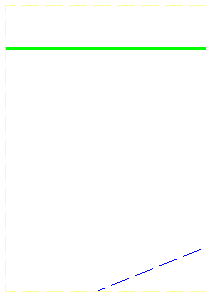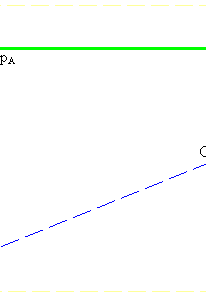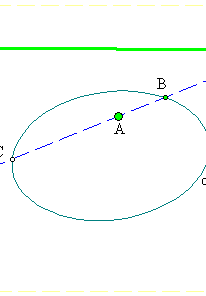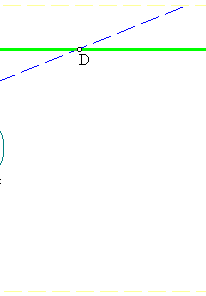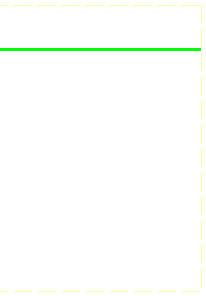The fact that the geometric locus described above is a line, can be seen in many ways. Two particularly easy are:
1) By deriving it through a projectivity from the corresponding property of the circle.
2) By using the equation of the conic (see Conic_Equation.html , section-5).
The figure below illustrates the usual way to draw the polar pA of a point A with respect to a conic c:
Draw two secants {BC, B'C'} through A and find the intersection points {E,E'} of opposite side-pairs (BC',CB') and (BB',CC').
Line EE' is the polar of A.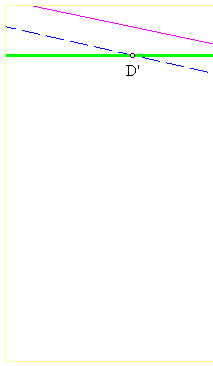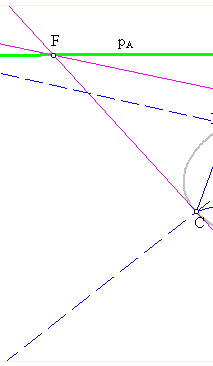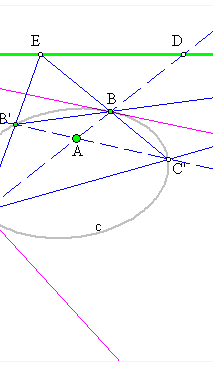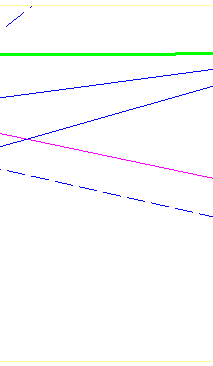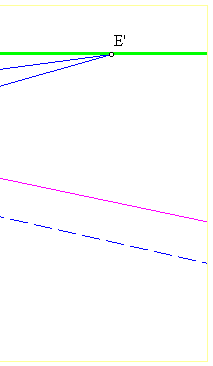The reason for this can be drawn back to Pascal's theorem (see Pascal.html or even better PascalOnQuadrangles.html ) by which the
tangents at {B',C'} concure also at a point F of line EE'. Then turning the secant B'C' about A we move D' on line pA while all the time
{B',C',A,D'} build a harmonic division. Line pA remains the same since it is the one passing from the immovable points D and F.

### See Also

Harmonic.html
Polar2.html
Polar3.html
Polar4.html
Conic_Equation.html
Pascal.html
PascalOnQuadrangles.html

Return to Gallery

 Produced with EucliDraw© http://users.math.uoc.gr/~pamfilos/